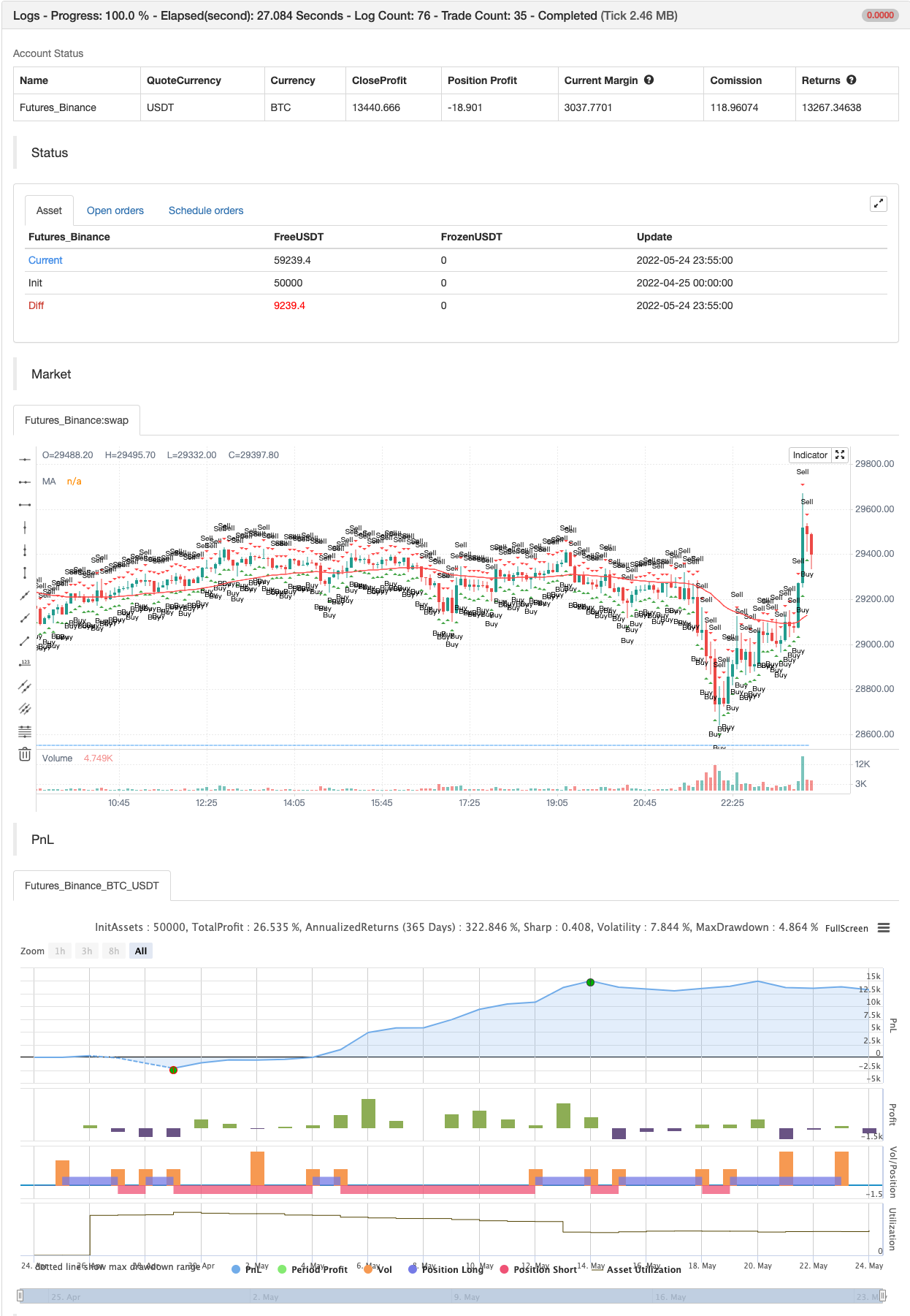Author: 张超, Date: 2022-05-26 17:11:01
Tags: ADX RMA RSI EMA sma WMA

This is a study indicator that shows the entries in the strategy seen in one of the youtube channel so it does not belong to me. I can’t tell who it is because it’s against the House Rules to advertise but you can find out if you look for it on youtube. Default values of oscilators and ema adjusted as suggested. He says he got the best results in 5 min timeframe but i tried to make things as modifiable as possible so you can mess around with the settings and create your own strategy for different timeframes if you’d like. Suggested to use with normal candlestick charts. The blue line below indicates the ADX is above the selected threshold set in the settings named “Trend Ready Limit”. You can set alerts for Buy, Sell or Buy/Sell signal together.

The entry strategy itself is pretty straight forward. The rules for entry are as follows, the script will check all of this on auto and will give you buy or sell signal: Recommended time frame: 5 min

For Long Entry:

• Check if price above the set EMA (Can disable this rule if you’d like in the settings)
• RSI is in Oversold
• ADX is above set “Trend Ready” threshold (Meaning there is a trend going on)
• Price must approve the trend of previous candles. This is bullish for buy entries and bearish for sell entries.
• Enter with stop loss below last swing low with 1:1 or 1.5:1 take profit ratio.

For Short Entry:

• Check if price below the set EMA (Can disable this rule if you’d like in the settings)
• RSI is in Overbought
• ADX is above set “Trend Ready” threshold (Meaning there is a trend going on)
• Price must approve the trend of previous candles. This is bullish for buy entries and bearish for sell entries.
• Enter with stop loss above last swing high with 1:1 or 1.5:1 take profit ratio.

This is my first indicator. Let me know if you want any updates. I am not sure if i can add everything but i’ll try nonetheless.

Changed: Signals will check up to 2 candles before if the RSI is below or above the set value to show signal. This is because sometimes the entry signal is right but the response might be a bit late.

backtest```/*backtest
start: 2022-04-25 00:00:00
end: 2022-05-24 23:59:00
period: 5m
basePeriod: 1m
exchanges: [{"eid":"Futures_Binance","currency":"BTC_USDT"}]
*/

//@version=5

//Define MA Inputs and group them
maType = input.string(title="MA Type", options=["EMA", "SMA", "WMA", "VWMA", "HMA", "RMA", "DEMA", "TEMA", "LSMA", "ZLSMA"], defval="EMA", group='MA Settings')
emaSource = input.source(title='MA Source', defval=close, group='MA Settings')
emaLength = input.int(title='MA Length', defval=50, minval=1, maxval=999, group='MA Settings')

//Other Moving Avarage Calculations
e1 = ta.ema(emaSource, emaLength)
e2 = ta.ema(e1, emaLength)
dema = 2 * e1 - e2

ema1 = ta.ema(emaSource, emaLength)
ema2 = ta.ema(ema1, emaLength)
ema3 = ta.ema(ema2, emaLength)
tema = 3 * (ema1 - ema2) + ema3

lsmaOffset = input.int(title="LSMA Offset", defval=0, minval=0, maxval=100, tooltip='Only used if you choose the LSMA and ZLSMA(Zero Lag LSMA) Option between MA Types', group='MA Settings')
lsma = ta.linreg(emaSource, emaLength, lsmaOffset)
lsma2 = ta.linreg(lsma, emaLength, lsmaOffset)
eq = lsma-lsma2
zlsma = lsma+eq

// Switch between different MA Types
emaValue = switch maType
"EMA" => ta.ema(emaSource, emaLength)
"SMA" => ta.sma(emaSource, emaLength)
"WMA" => ta.wma(emaSource, emaLength)
"VWMA" => ta.vwma(emaSource, emaLength)
"HMA" => ta.hma(emaSource, emaLength)
"RMA" => ta.rma(emaSource, emaLength)
"DEMA" => dema
"TEMA" => tema
"LSMA" => lsma
"ZLSMA" => zlsma
=>
runtime.error("No matching MA type found.")
float(na)

//Define RSI inputs and group them
rsiSource = input.source(title='RSI Source', defval=close, group='RSI Settings')
rsiLength = input.int(title='RSI Length', defval=3, minval=0, maxval=100, group='RSI Settings')
rsiValuee = ta.rsi(rsiSource, rsiLength)
rsiOverbought = input.int(title='RSI Overbought Level', defval=80, group='RSI Settings')
rsiOversold = input.int(title='RSI Oversold Level', defval=20, group='RSI Settings')

//Define overbought and oversold conditions
isRsiOB = rsiValuee >= rsiOverbought
isRsiOS = rsiValuee <= rsiOversold

//ADX Inputs and calculation of the value
dilen = input.int(5, title='DI Length', group='ADX Settings')
dirmov(len) =>
up = ta.change(high)
down = -ta.change(low)
plusDM = na(up) ? na : up > down and up > 0 ? up : 0
minusDM = na(down) ? na : down > up and down > 0 ? down : 0
truerange = ta.rma(ta.tr, len)
plus = fixnan(100 * ta.rma(plusDM, len) / truerange)
minus = fixnan(100 * ta.rma(minusDM, len) / truerange)
[plus, minus]
[plus, minus] = dirmov(dilen)
sum = plus + minus
adx = 100 * ta.rma(math.abs(plus - minus) / (sum == 0 ? 1 : sum), adxlen)

//Define the input and value where it is considered that there is a trend going on

//Draw trend ready at the bottom of the chart for better viewing so that you can change the value based on what you see easier

//Plot the EMA on chart
enableEmaRule = input(title='Enable MA Rule', defval=true)

//Define the signal conditions and choice to add or leave out MA Rule if you wish so
alertLong = enableEmaRule ? low > emaValue and (rsiValuee <= rsiOversold or rsiValuee <= rsiOversold or rsiValuee <= rsiOversold) and sig > adxLimit and close > high : (rsiValuee <= rsiOversold or rsiValuee <= rsiOversold or rsiValuee <= rsiOversold) and sig > adxLimit and close > high
alertShort = enableEmaRule ? high < emaValue and (rsiValuee >= rsiOverbought or rsiValuee >= rsiOverbought or rsiValuee >= rsiOverbought) and sig > adxLimit and close < low : (rsiValuee >= rsiOverbought or rsiValuee >= rsiOverbought or rsiValuee >= rsiOverbought) and sig > adxLimit and close < low
plot(enableEmaRule ? emaValue : na, color=color.new(color.red, 0), title='MA')

//Buy and Sell Shapes on Chart
plotshape(alertShort, title='Sell', location=location.abovebar, color=color.new(color.red, 0), size=size.small, style=shape.triangledown, text='Sell')